Скачать презентацию Chapter 8 Annual Equivalent Worth Analysis Annual

c5a093a45882ed96af4194806f4044f0.ppt

• Количество слайдов: 38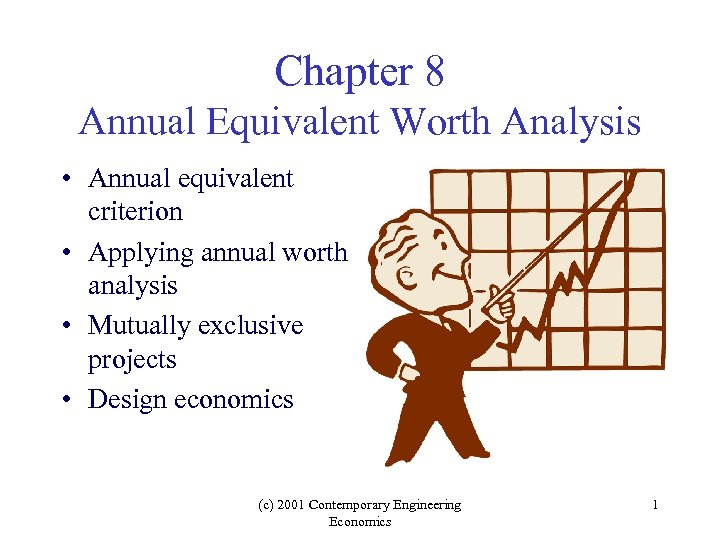Chapter 8 Annual Equivalent Worth Analysis • Annual equivalent criterion • Applying annual worth analysis • Mutually exclusive projects • Design economics (c) 2001 Contemporary Engineering Economics 1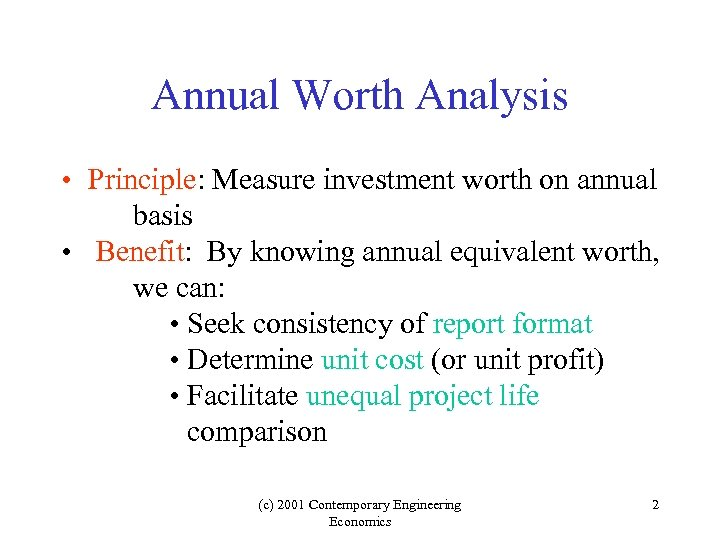Annual Worth Analysis • Principle: Measure investment worth on annual basis • Benefit: By knowing annual equivalent worth, we can: • Seek consistency of report format • Determine unit cost (or unit profit) • Facilitate unequal project life comparison (c) 2001 Contemporary Engineering Economics 2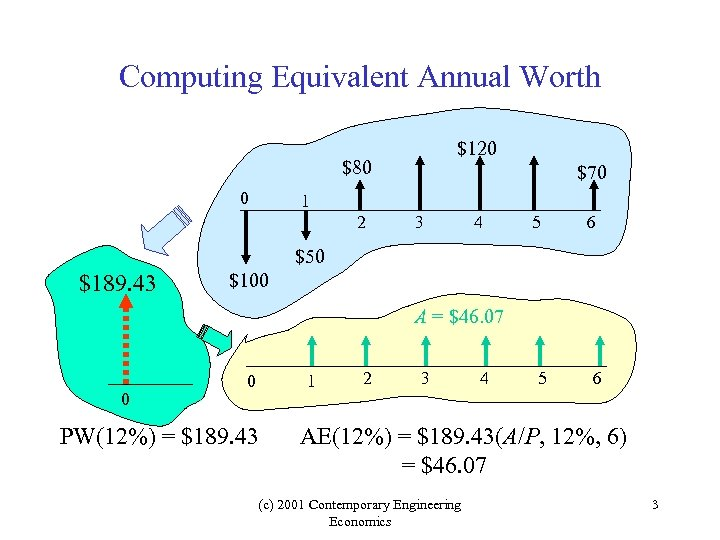Computing Equivalent Annual Worth \$120 \$80 0 \$70 1 2 3 4 5 6 \$50 \$189. 43 \$100 A = \$46. 07 0 0 1 PW(12%) = \$189. 43 2 3 4 5 6 AE(12%) = \$189. 43(A/P, 12%, 6) = \$46. 07 (c) 2001 Contemporary Engineering Economics 3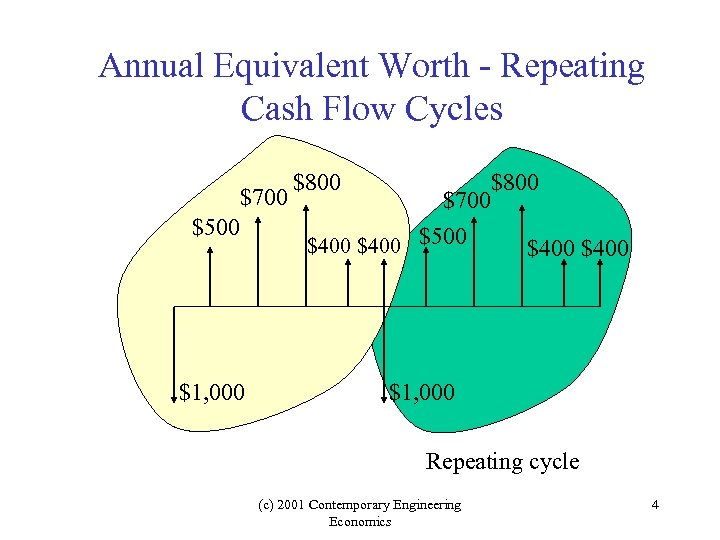Annual Equivalent Worth - Repeating Cash Flow Cycles \$700 \$500 \$1, 000 \$800 \$700 \$400 \$500 \$400 \$1, 000 Repeating cycle (c) 2001 Contemporary Engineering Economics 4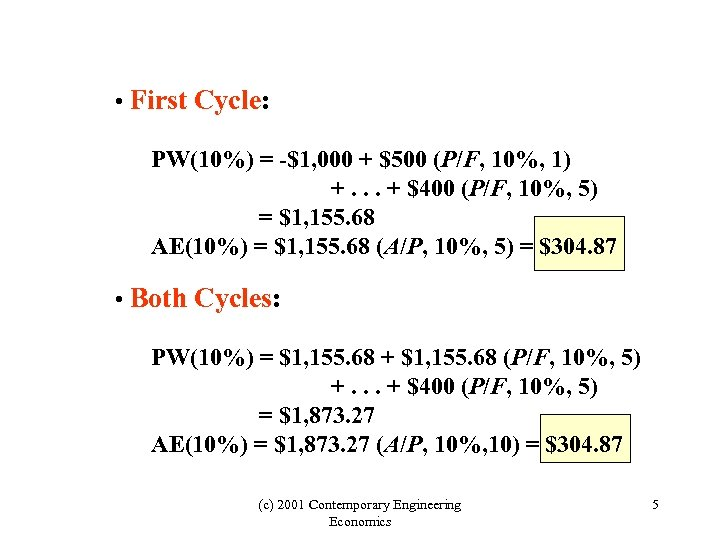• First Cycle: PW(10%) = -\$1, 000 + \$500 (P/F, 10%, 1) +. . . + \$400 (P/F, 10%, 5) = \$1, 155. 68 AE(10%) = \$1, 155. 68 (A/P, 10%, 5) = \$304. 87 • Both Cycles: PW(10%) = \$1, 155. 68 + \$1, 155. 68 (P/F, 10%, 5) +. . . + \$400 (P/F, 10%, 5) = \$1, 873. 27 AE(10%) = \$1, 873. 27 (A/P, 10%, 10) = \$304. 87 (c) 2001 Contemporary Engineering Economics 5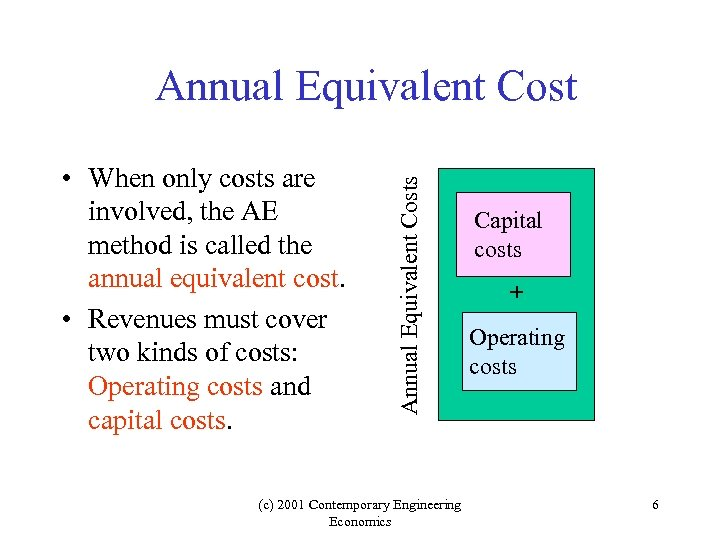• When only costs are involved, the AE method is called the annual equivalent cost. • Revenues must cover two kinds of costs: Operating costs and capital costs. Annual Equivalent Costs Annual Equivalent Cost (c) 2001 Contemporary Engineering Economics Capital costs + Operating costs 6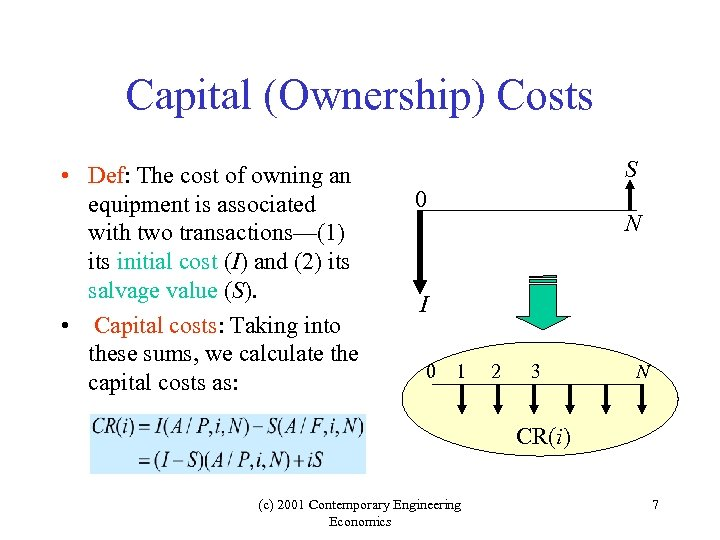Capital (Ownership) Costs • Def: The cost of owning an equipment is associated with two transactions—(1) its initial cost (I) and (2) its salvage value (S). • Capital costs: Taking into these sums, we calculate the capital costs as: S 0 N I 0 1 2 3 N CR(i) (c) 2001 Contemporary Engineering Economics 7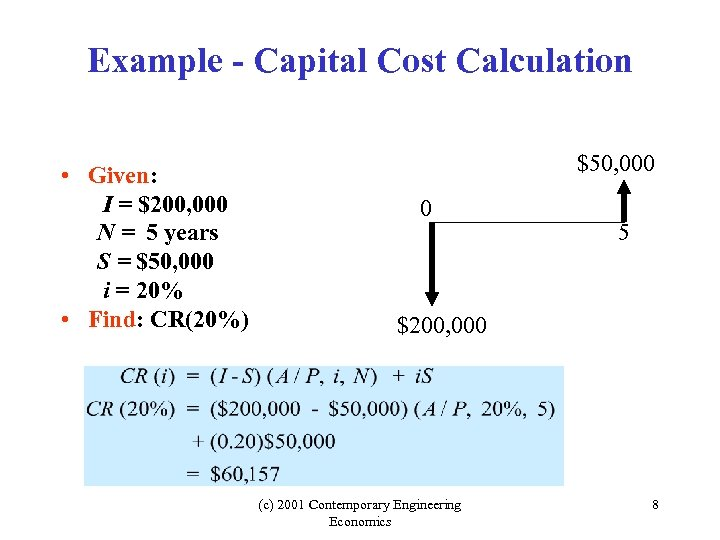Example - Capital Cost Calculation • Given: I = \$200, 000 N = 5 years S = \$50, 000 i = 20% • Find: CR(20%) \$50, 000 0 5 \$200, 000 (c) 2001 Contemporary Engineering Economics 8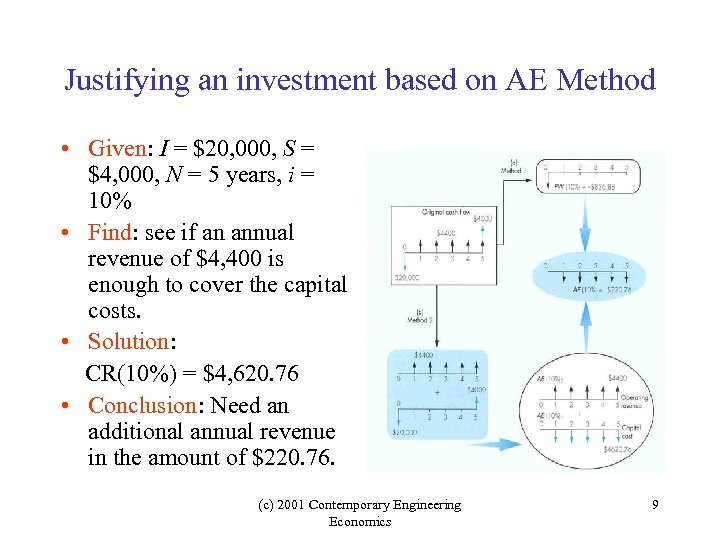Justifying an investment based on AE Method • Given: I = \$20, 000, S = \$4, 000, N = 5 years, i = 10% • Find: see if an annual revenue of \$4, 400 is enough to cover the capital costs. • Solution: CR(10%) = \$4, 620. 76 • Conclusion: Need an additional annual revenue in the amount of \$220. 76. (c) 2001 Contemporary Engineering Economics 9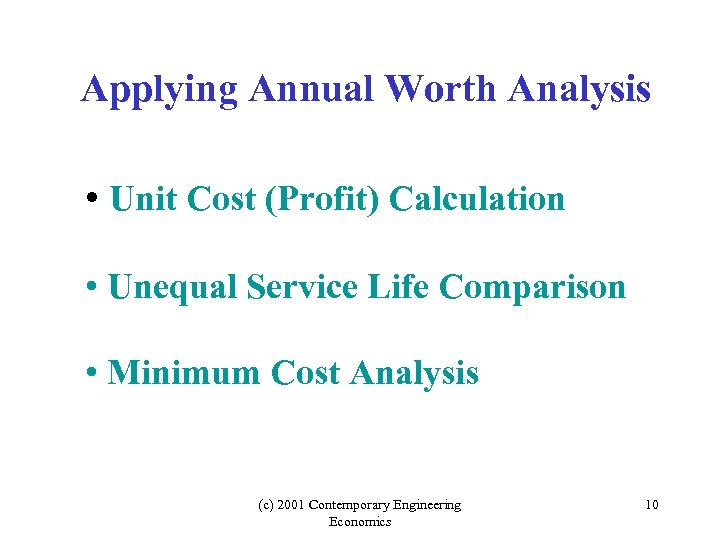Applying Annual Worth Analysis • Unit Cost (Profit) Calculation • Unequal Service Life Comparison • Minimum Cost Analysis (c) 2001 Contemporary Engineering Economics 10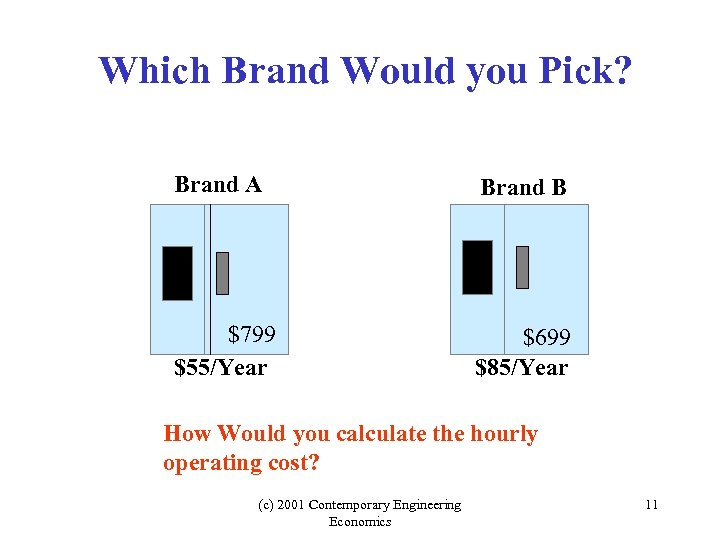Which Brand Would you Pick? Brand A Brand B \$799 \$55/Year \$699 \$85/Year How Would you calculate the hourly operating cost? (c) 2001 Contemporary Engineering Economics 11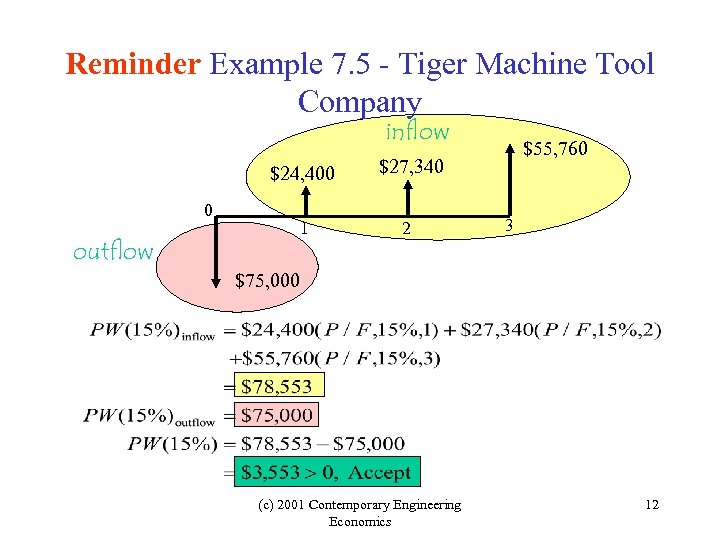Reminder Example 7. 5 - Tiger Machine Tool Company inflow \$24, 400 0 outflow \$27, 340 1 2 \$55, 760 3 \$75, 000 (c) 2001 Contemporary Engineering Economics 12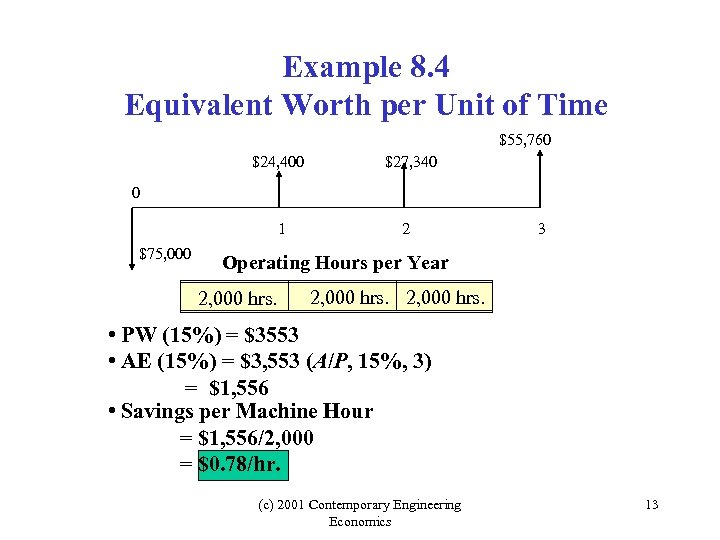Example 8. 4 Equivalent Worth per Unit of Time \$55, 760 \$24, 400 \$27, 340 1 2 0 \$75, 000 3 Operating Hours per Year 2, 000 hrs. • PW (15%) = \$3553 • AE (15%) = \$3, 553 (A/P, 15%, 3) = \$1, 556 • Savings per Machine Hour = \$1, 556/2, 000 = \$0. 78/hr. (c) 2001 Contemporary Engineering Economics 13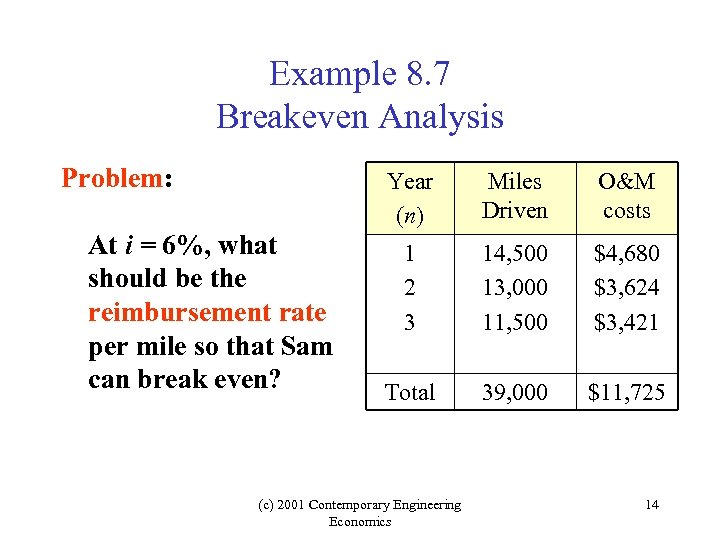Example 8. 7 Breakeven Analysis Problem: Year (n) At i = 6%, what should be the reimbursement rate per mile so that Sam can break even? Miles Driven O&M costs 1 2 3 14, 500 13, 000 11, 500 \$4, 680 \$3, 624 \$3, 421 Total 39, 000 \$11, 725 (c) 2001 Contemporary Engineering Economics 14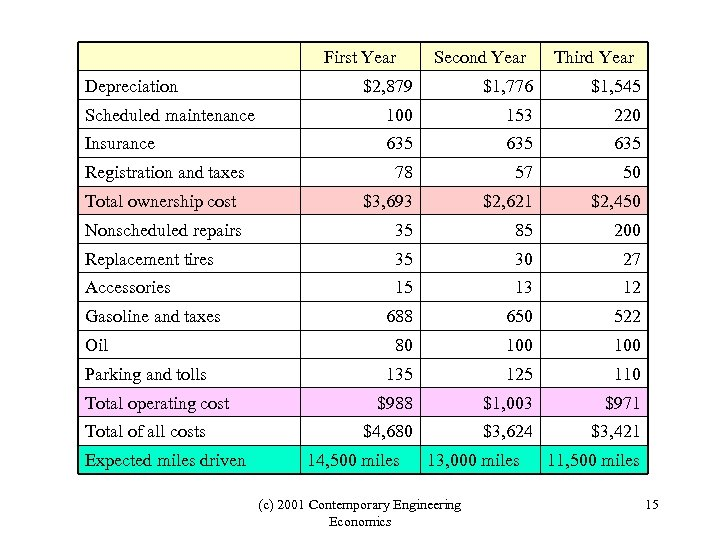First Year Depreciation Second Year Third Year \$2, 879 \$1, 776 \$1, 545 Scheduled maintenance 100 153 220 Insurance 635 635 78 57 50 \$3, 693 \$2, 621 \$2, 450 Nonscheduled repairs 35 85 200 Replacement tires 35 30 27 Accessories 15 13 12 688 650 522 80 100 135 125 110 \$988 \$1, 003 \$971 \$4, 680 \$3, 624 \$3, 421 Registration and taxes Total ownership cost Gasoline and taxes Oil Parking and tolls Total operating cost Total of all costs Expected miles driven 14, 500 miles 13, 000 miles (c) 2001 Contemporary Engineering Economics 11, 500 miles 15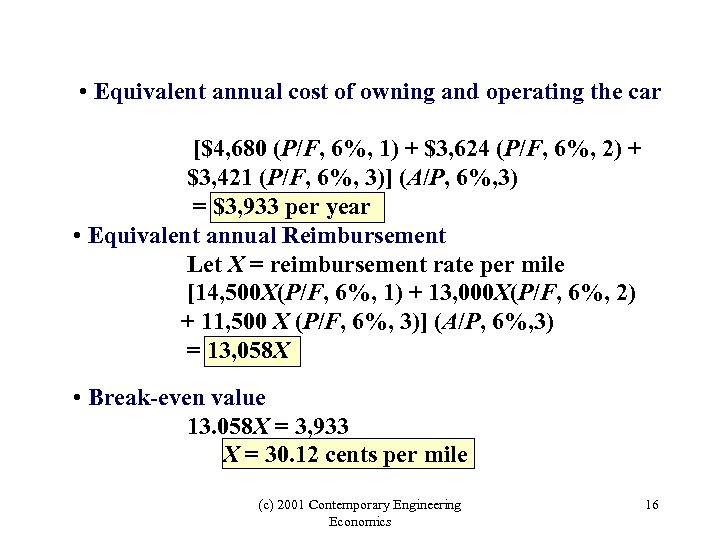• Equivalent annual cost of owning and operating the car [\$4, 680 (P/F, 6%, 1) + \$3, 624 (P/F, 6%, 2) + \$3, 421 (P/F, 6%, 3)] (A/P, 6%, 3) = \$3, 933 per year • Equivalent annual Reimbursement Let X = reimbursement rate per mile [14, 500 X(P/F, 6%, 1) + 13, 000 X(P/F, 6%, 2) + 11, 500 X (P/F, 6%, 3)] (A/P, 6%, 3) = 13, 058 X • Break-even value 13. 058 X = 3, 933 X = 30. 12 cents per mile (c) 2001 Contemporary Engineering Economics 16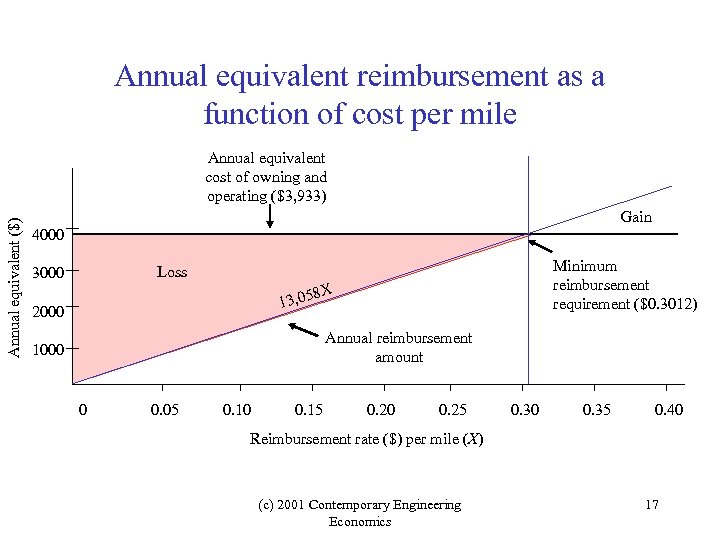Annual equivalent reimbursement as a function of cost per mile Annual equivalent (\$) Annual equivalent cost of owning and operating (\$3, 933) Gain 4000 Minimum reimbursement requirement (\$0. 3012) Loss 3000 8 X 13, 05 2000 Annual reimbursement amount 1000 0 0. 05 0. 10 0. 15 0. 20 0. 25 0. 30 0. 35 0. 40 Reimbursement rate (\$) per mile (X) (c) 2001 Contemporary Engineering Economics 17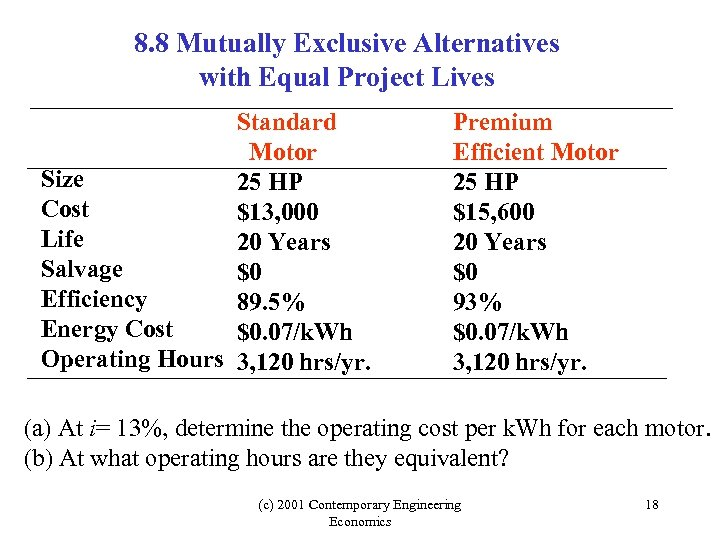8. 8 Mutually Exclusive Alternatives with Equal Project Lives Standard Motor Size 25 HP Cost \$13, 000 Life 20 Years Salvage \$0 Efficiency 89. 5% Energy Cost \$0. 07/k. Wh Operating Hours 3, 120 hrs/yr. Premium Efficient Motor 25 HP \$15, 600 20 Years \$0 93% \$0. 07/k. Wh 3, 120 hrs/yr. (a) At i= 13%, determine the operating cost per k. Wh for each motor. (b) At what operating hours are they equivalent? (c) 2001 Contemporary Engineering Economics 18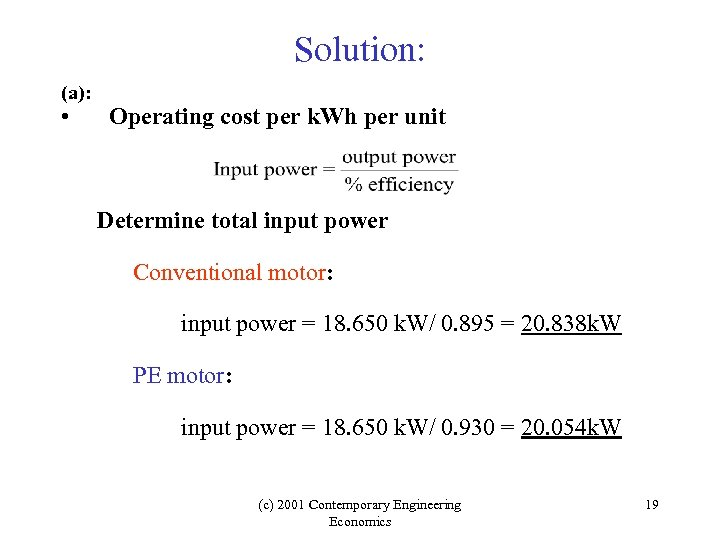Solution: (a): • Operating cost per k. Wh per unit Determine total input power Conventional motor: input power = 18. 650 k. W/ 0. 895 = 20. 838 k. W PE motor: input power = 18. 650 k. W/ 0. 930 = 20. 054 k. W (c) 2001 Contemporary Engineering Economics 19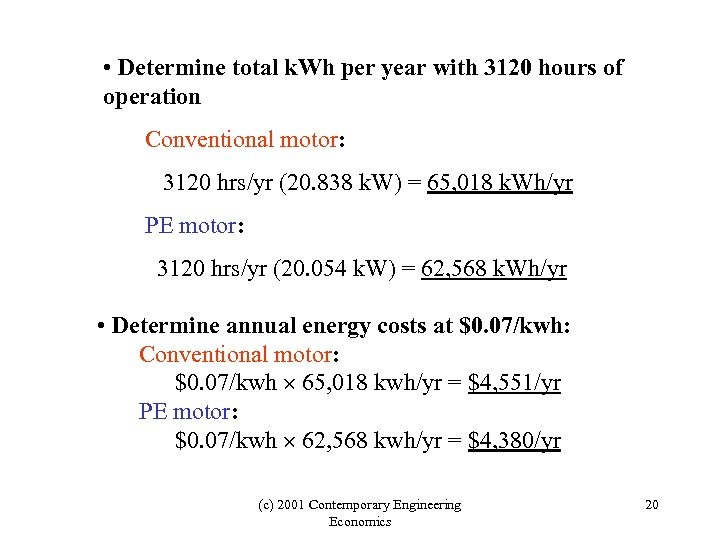• Determine total k. Wh per year with 3120 hours of operation Conventional motor: 3120 hrs/yr (20. 838 k. W) = 65, 018 k. Wh/yr PE motor: 3120 hrs/yr (20. 054 k. W) = 62, 568 k. Wh/yr • Determine annual energy costs at \$0. 07/kwh: Conventional motor: \$0. 07/kwh 65, 018 kwh/yr = \$4, 551/yr PE motor: \$0. 07/kwh 62, 568 kwh/yr = \$4, 380/yr (c) 2001 Contemporary Engineering Economics 20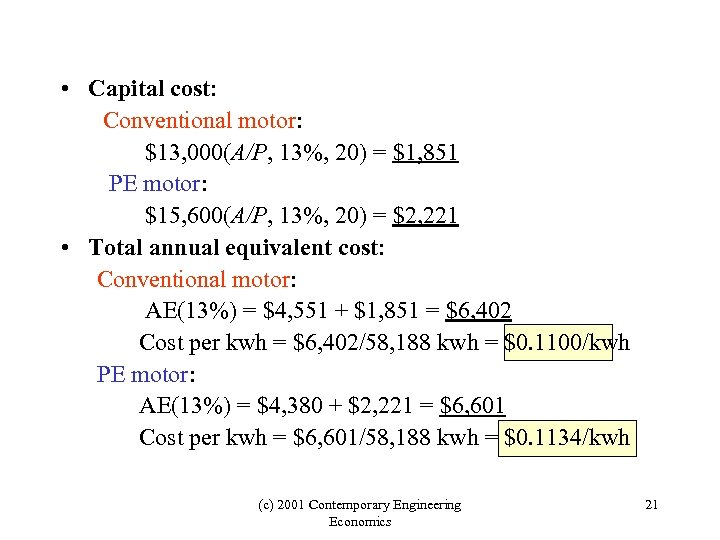• Capital cost: Conventional motor: \$13, 000(A/P, 13%, 20) = \$1, 851 PE motor: \$15, 600(A/P, 13%, 20) = \$2, 221 • Total annual equivalent cost: Conventional motor: AE(13%) = \$4, 551 + \$1, 851 = \$6, 402 Cost per kwh = \$6, 402/58, 188 kwh = \$0. 1100/kwh PE motor: AE(13%) = \$4, 380 + \$2, 221 = \$6, 601 Cost per kwh = \$6, 601/58, 188 kwh = \$0. 1134/kwh (c) 2001 Contemporary Engineering Economics 21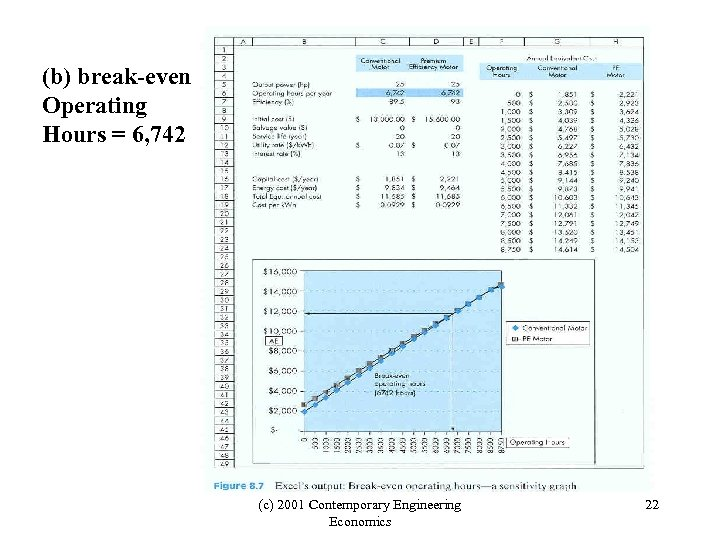(b) break-even Operating Hours = 6, 742 (c) 2001 Contemporary Engineering Economics 22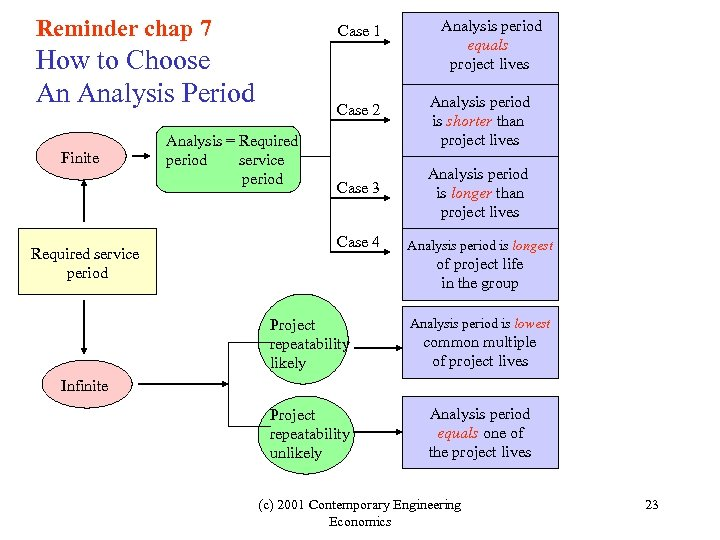Reminder chap 7 Case 1 How to Choose An Analysis Period Finite Required service period Case 2 Analysis = Required period service period Analysis period equals project lives Analysis period is shorter than project lives Case 3 Analysis period is longer than project lives Case 4 Analysis period is longest of project life in the group Project repeatability likely Analysis period is lowest Project repeatability unlikely Analysis period equals one of the project lives common multiple of project lives Infinite (c) 2001 Contemporary Engineering Economics 23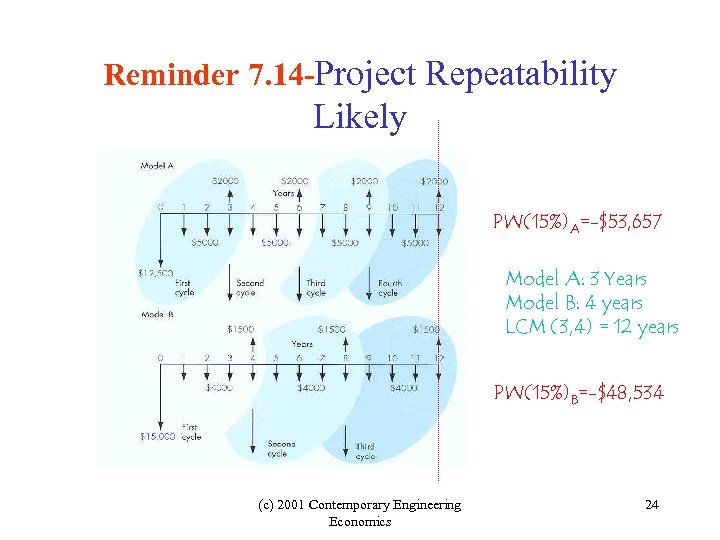Reminder 7. 14 -Project Repeatability Likely PW(15%)A=-\$53, 657 Model A: 3 Years Model B: 4 years LCM (3, 4) = 12 years PW(15%)B=-\$48, 534 (c) 2001 Contemporary Engineering Economics 24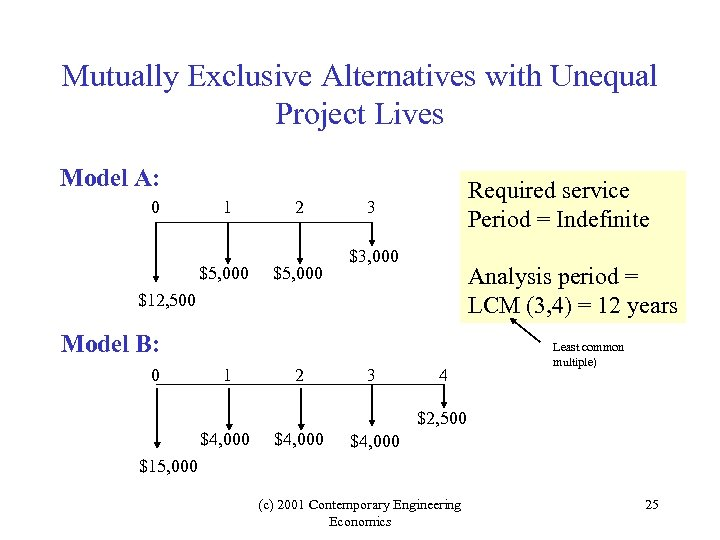Mutually Exclusive Alternatives with Unequal Project Lives Model A: 0 1 \$5, 000 2 \$5, 000 Required service Period = Indefinite 3 \$3, 000 Analysis period = LCM (3, 4) = 12 years \$12, 500 Model B: 0 1 2 3 4 Least common multiple) \$2, 500 \$4, 000 \$15, 000 (c) 2001 Contemporary Engineering Economics 25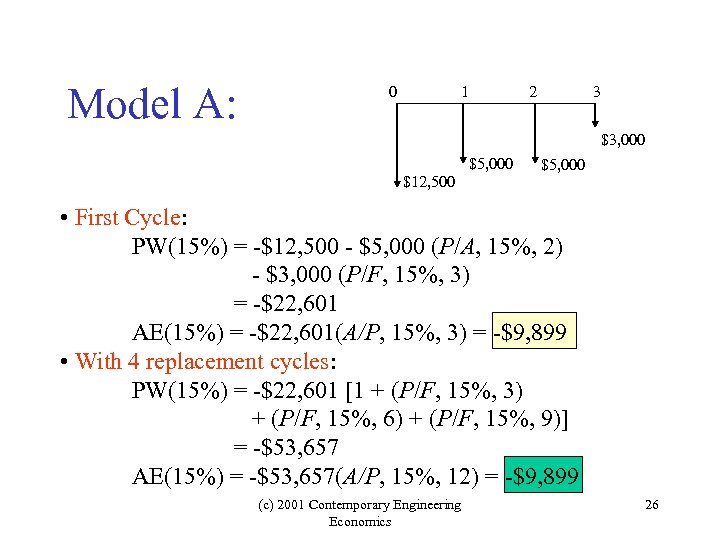Model A: 0 1 2 3 \$3, 000 \$5, 000 \$12, 500 \$5, 000 • First Cycle: PW(15%) = -\$12, 500 - \$5, 000 (P/A, 15%, 2) - \$3, 000 (P/F, 15%, 3) = -\$22, 601 AE(15%) = -\$22, 601(A/P, 15%, 3) = -\$9, 899 • With 4 replacement cycles: PW(15%) = -\$22, 601 [1 + (P/F, 15%, 3) + (P/F, 15%, 6) + (P/F, 15%, 9)] = -\$53, 657 AE(15%) = -\$53, 657(A/P, 15%, 12) = -\$9, 899 (c) 2001 Contemporary Engineering Economics 26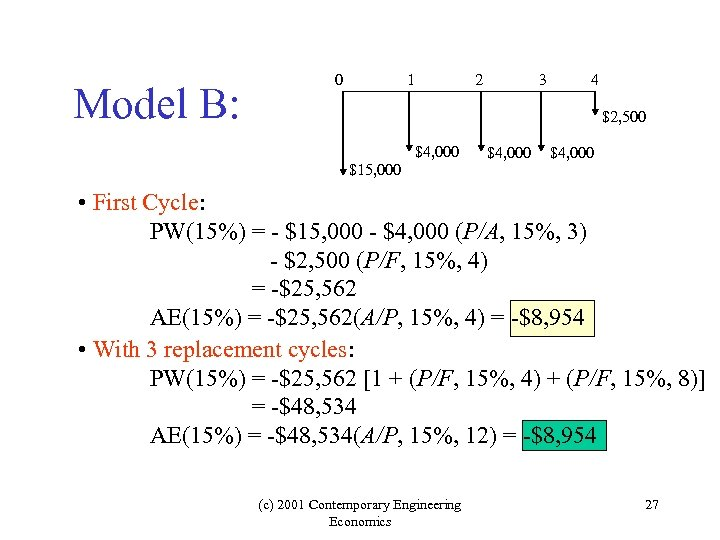Model B: 0 1 2 3 4 \$2, 500 \$4, 000 \$15, 000 \$4, 000 • First Cycle: PW(15%) = - \$15, 000 - \$4, 000 (P/A, 15%, 3) - \$2, 500 (P/F, 15%, 4) = -\$25, 562 AE(15%) = -\$25, 562(A/P, 15%, 4) = -\$8, 954 • With 3 replacement cycles: PW(15%) = -\$25, 562 [1 + (P/F, 15%, 4) + (P/F, 15%, 8)] = -\$48, 534 AE(15%) = -\$48, 534(A/P, 15%, 12) = -\$8, 954 (c) 2001 Contemporary Engineering Economics 27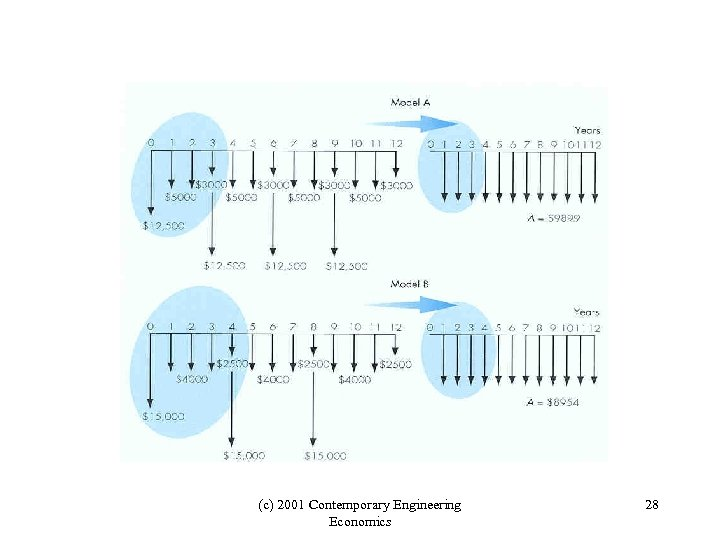(c) 2001 Contemporary Engineering Economics 28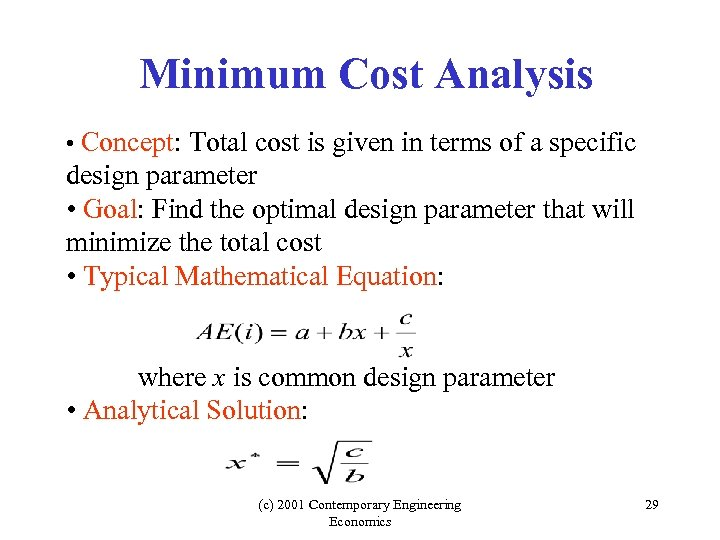Minimum Cost Analysis • Concept: Total cost is given in terms of a specific design parameter • Goal: Find the optimal design parameter that will minimize the total cost • Typical Mathematical Equation: where x is common design parameter • Analytical Solution: (c) 2001 Contemporary Engineering Economics 29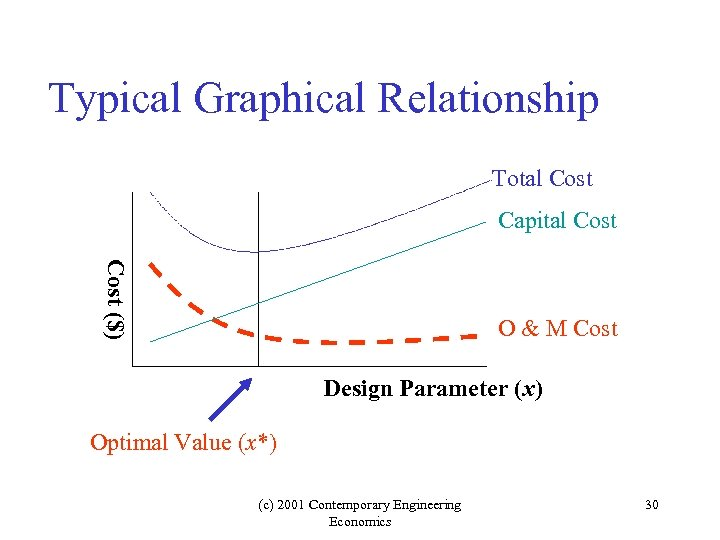Typical Graphical Relationship Total Cost Capital Cost (\$) O & M Cost Design Parameter (x) Optimal Value (x*) (c) 2001 Contemporary Engineering Economics 30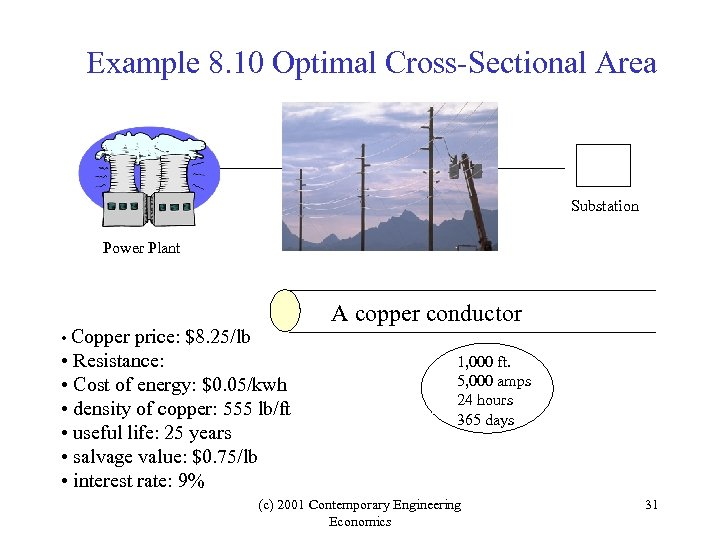Example 8. 10 Optimal Cross-Sectional Area Substation Power Plant • Copper price: \$8. 25/lb • Resistance: • Cost of energy: \$0. 05/kwh • density of copper: 555 lb/ft • useful life: 25 years • salvage value: \$0. 75/lb • interest rate: 9% A copper conductor 1, 000 ft. 5, 000 amps 24 hours 365 days (c) 2001 Contemporary Engineering Economics 31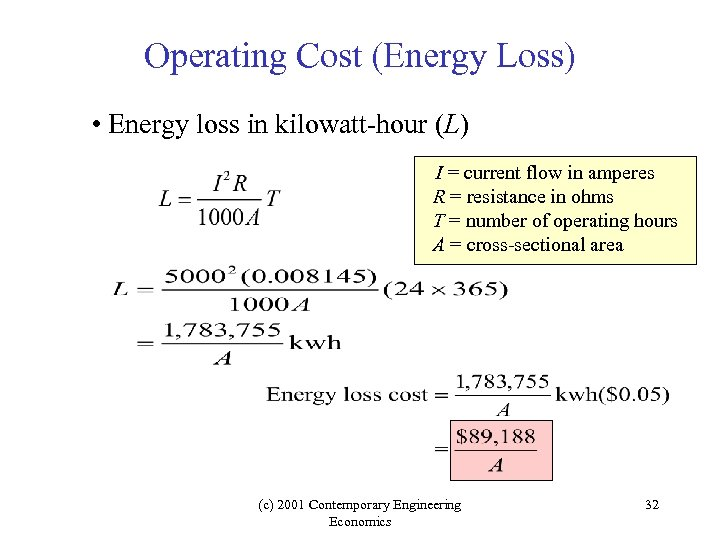Operating Cost (Energy Loss) • Energy loss in kilowatt-hour (L) I = current flow in amperes R = resistance in ohms T = number of operating hours A = cross-sectional area (c) 2001 Contemporary Engineering Economics 32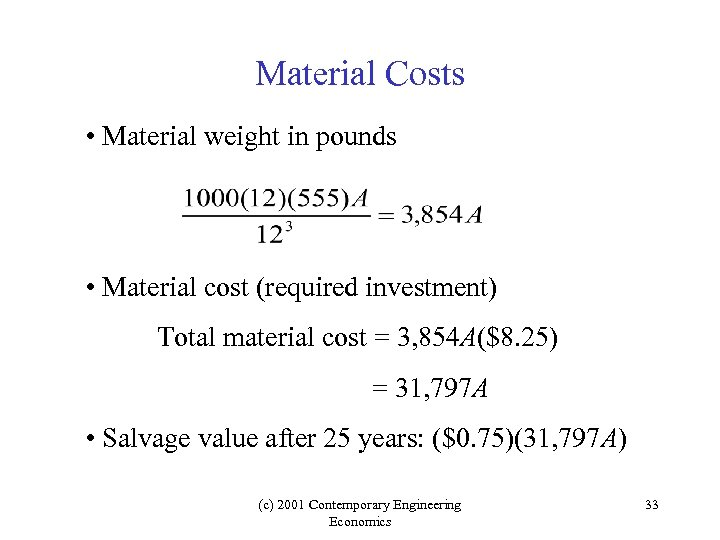Material Costs • Material weight in pounds • Material cost (required investment) Total material cost = 3, 854 A(\$8. 25) = 31, 797 A • Salvage value after 25 years: (\$0. 75)(31, 797 A) (c) 2001 Contemporary Engineering Economics 33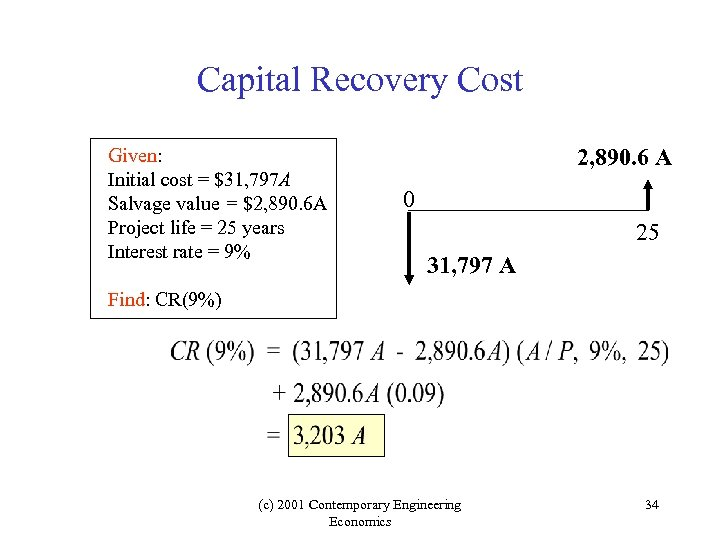Capital Recovery Cost Given: Initial cost = \$31, 797 A Salvage value = \$2, 890. 6 A Project life = 25 years Interest rate = 9% 2, 890. 6 A 0 25 31, 797 A Find: CR(9%) (c) 2001 Contemporary Engineering Economics 34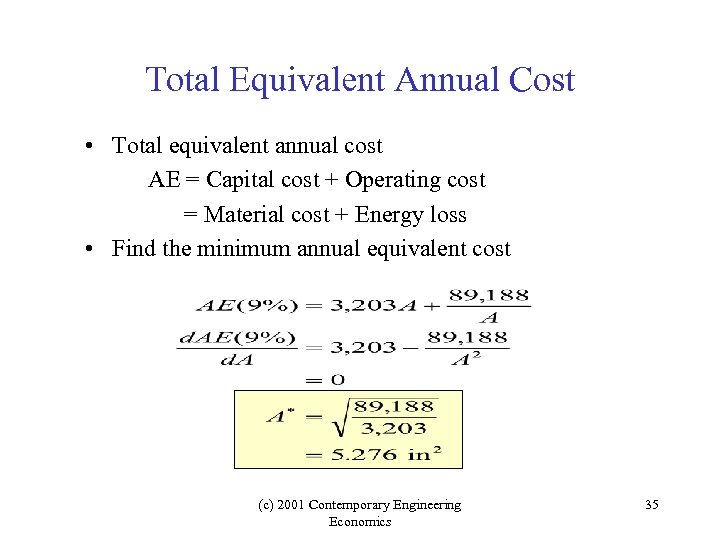Total Equivalent Annual Cost • Total equivalent annual cost AE = Capital cost + Operating cost = Material cost + Energy loss • Find the minimum annual equivalent cost (c) 2001 Contemporary Engineering Economics 35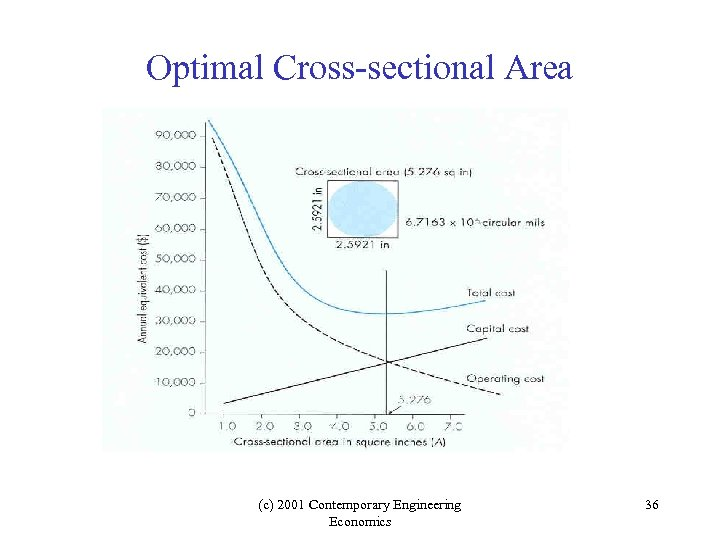Optimal Cross-sectional Area (c) 2001 Contemporary Engineering Economics 36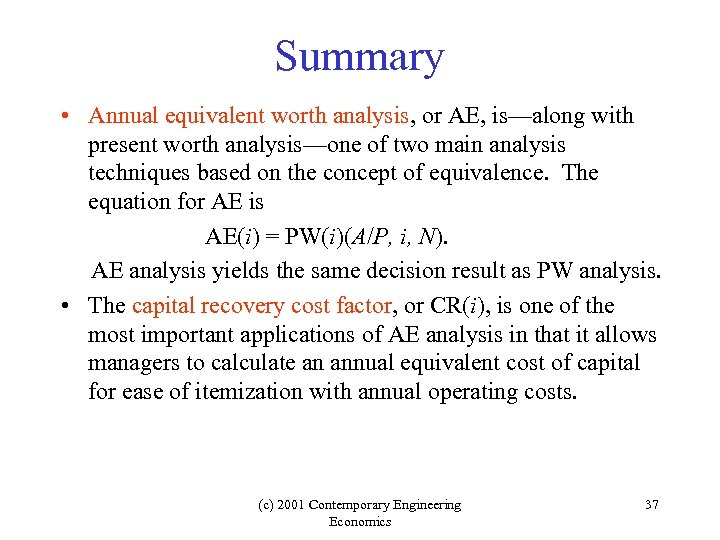Summary • Annual equivalent worth analysis, or AE, is—along with present worth analysis—one of two main analysis techniques based on the concept of equivalence. The equation for AE is AE(i) = PW(i)(A/P, i, N). AE analysis yields the same decision result as PW analysis. • The capital recovery cost factor, or CR(i), is one of the most important applications of AE analysis in that it allows managers to calculate an annual equivalent cost of capital for ease of itemization with annual operating costs. (c) 2001 Contemporary Engineering Economics 37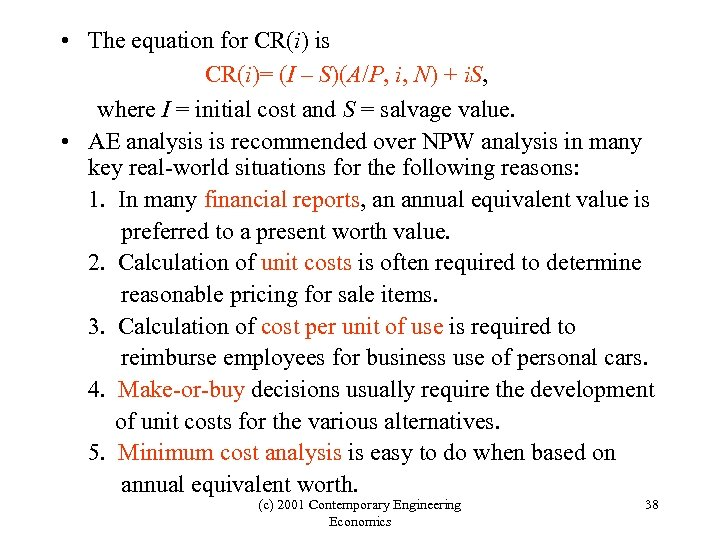• The equation for CR(i) is CR(i)= (I – S)(A/P, i, N) + i. S, where I = initial cost and S = salvage value. • AE analysis is recommended over NPW analysis in many key real-world situations for the following reasons: 1. In many financial reports, an annual equivalent value is preferred to a present worth value. 2. Calculation of unit costs is often required to determine reasonable pricing for sale items. 3. Calculation of cost per unit of use is required to reimburse employees for business use of personal cars. 4. Make-or-buy decisions usually require the development of unit costs for the various alternatives. 5. Minimum cost analysis is easy to do when based on annual equivalent worth. (c) 2001 Contemporary Engineering Economics 38